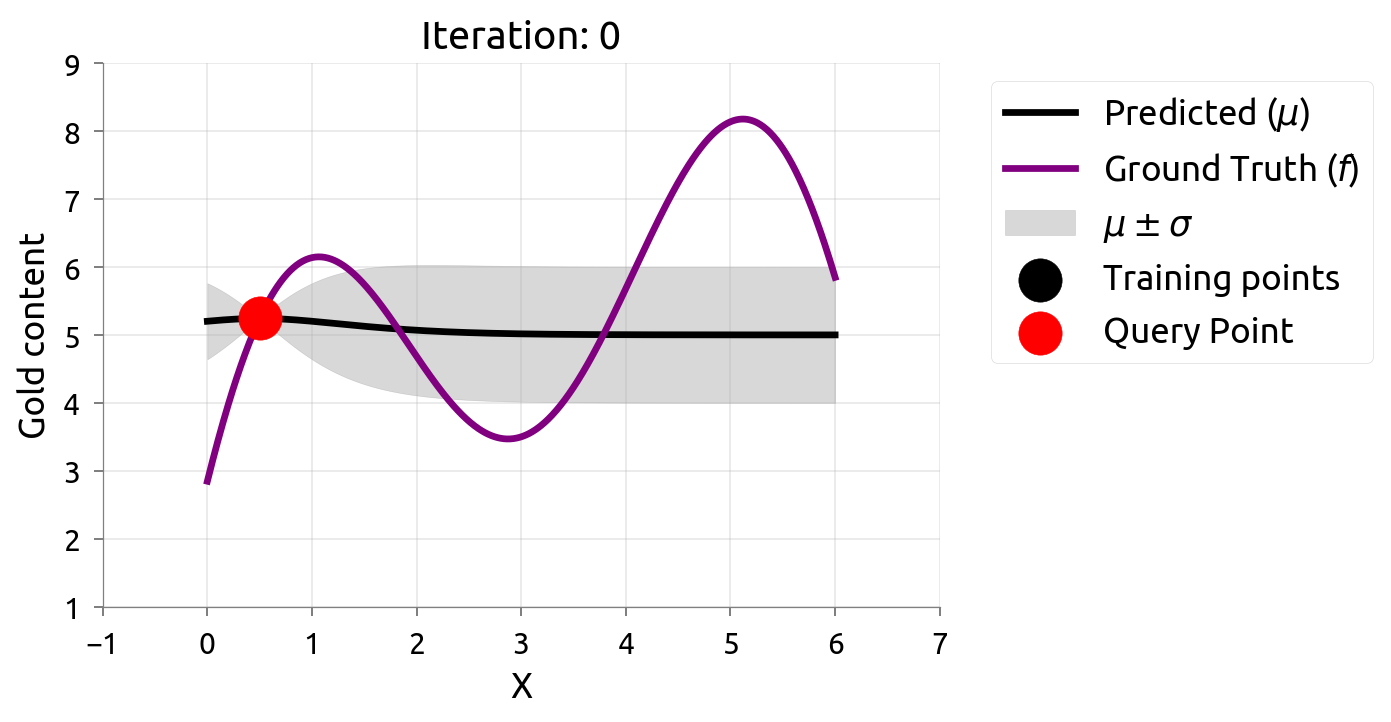# 高斯分布

## 一元高斯分布

$$X \sim N \left(\mu, \sigma^2\right)$$

$$f \left(x\right) = \dfrac{1}{\sigma \sqrt{2 \pi}} e^{- \dfrac{\left(x - \mu\right)^2}{2 \sigma^2}}$$

## 二元高斯分布

$$\left(X, Y\right) \sim \mathcal{N} \left(\mu, \sigma\right)$$

$$f(x, y)=\frac{1}{2 \pi \sigma_{X} \sigma_{Y} \sqrt{1-\rho^{2}}} e^{-\dfrac{1}{2\left(1-\rho^{2}\right)}\left[\dfrac{\left(x-\mu_{X}\right)^{2}}{\sigma_{X}^{2}}+\dfrac{\left(y-\mu_{Y}\right)^{2}}{\sigma_{Y}^{2}}-\dfrac{2 \rho\left(x-\mu_{X}\right)\left(y-\mu_{X}\right)}{\sigma_{X} \sigma_{Y}}\right]}$$

## 多元高斯分布

$K$ 维随机向量 $X = \left[X_1, \cdots, X_K\right]^{\top}$ 服从多元高斯分布，则必须满足如下三个等价条件：

1. 任何线性组合 $Y = a_1 X_1 + \cdots a_K X_K$ 均服从高斯分布。
2. 存在随机向量 $Z = \left[Z_1, \cdots, Z_L\right]^{\top}$（每个元素服从独立标准高斯分布），向量 $\mu = \left[\mu_1, \cdots, \mu_K\right]^{\top}$ 以及 $K \times L$ 的矩阵 $A$，满足 $X = A Z + \mu$
3. 存在 $\mu$ 和一个对称半正定矩阵 $\Sigma$ 满足 $X$ 的特征函数 $\phi_X \left(u; \mu, \Sigma\right) = \exp \left(i \mu^{\top} u - \dfrac{1}{2} u^{\top} \Sigma u\right)$

$$f \left(x_1, \cdots, x_k\right) = \dfrac{1}{\sqrt{\left(2 \pi\right)^k \lvert\Sigma\rvert}} e^{- \dfrac{1}{2} \left(x - \mu\right)^{\top} \Sigma^{-1} \left(x - \mu\right)}$$

## 边缘化和条件化

$$P_{X, Y}=\left[\begin{array}{l} X \\ Y \end{array}\right] \sim \mathcal{N}(\mu, \Sigma)=\mathcal{N}\left(\left[\begin{array}{l} \mu_{X} \\ \mu_{Y} \end{array}\right],\left[\begin{array}{l} \Sigma_{X X} \Sigma_{X Y} \\ \Sigma_{Y X} \Sigma_{Y Y} \end{array}\right]\right)$$

$$\begin{array}{l} X \sim \mathcal{N}\left(\mu_{X}, \Sigma_{X X}\right) \\ Y \sim \mathcal{N}\left(\mu_{Y}, \Sigma_{Y Y}\right) \end{array}$$

$X$$Y$ 两个子集各自只依赖于 $\mu$$\Sigma$ 中它们对应的值。因此从高斯分布中边缘化一个随机变量仅需从 $\mu$$\Sigma$ 中舍弃相应的变量即可：

$$p_{X}(x)=\int_{y} p_{X, Y}(x, y) d y=\int_{y} p_{X | Y}(x | y) p_{Y}(y) d y$$

$$\begin{array}{l} X | Y \sim \mathcal{N}\left(\mu_{X}+\Sigma_{X Y} \Sigma_{Y Y}^{-1}\left(Y-\mu_{Y}\right), \Sigma_{X X}-\Sigma_{X Y} \Sigma_{Y Y}^{-1} \Sigma_{Y X}\right) \\ Y | X \sim \mathcal{N}\left(\mu_{Y}+\Sigma_{Y X} \Sigma_{X X}^{-1}\left(X-\mu_{X}\right), \Sigma_{Y Y}-\Sigma_{Y X} \Sigma_{X X}^{-1} \Sigma_{X Y}\right) \end{array}$$

# 高斯过程

**高斯过程（Gaussian Process）**是观测值出现在一个连续域（例如时间或空间）的随机过程。在高斯过程中，连续输入空间中每个点都是与一个正态分布的随机变量相关联。此外，这些随机变量的每个有限集合都有一个多元正态分布，换句话说它们的任意有限线性组合是一个正态分布。高斯过程的分布是所有那些（无限多个）随机变量的联合分布，正因如此，它是连续域（例如时间或空间）上函数的分布。

\begin{aligned} m(x) &=\mathbb{E}[f(x)] \\ K\left(x, x'\right) &=\mathbb{E}\left[(f(x)-m(x))\left(f\left(x^{\prime}\right)-m\left(x^{\prime}\right)\right)\right] \end{aligned}

$$f \left(x\right) \sim \mathcal{GP} \left(m \left(x\right), K \left(x, x'\right)\right)$$

• 常数：$K_c \left(x, x'\right) = C$
• 线性：$K_L \left(x, x'\right) = x^{\top} x'$
• 高斯噪声：$K_{GN} \left(x, x'\right) = \sigma^2 \delta_{x, x'}$
• 指数平方：$K_{\mathrm{SE}}\left(x, x^{\prime}\right)=\exp \left(-\dfrac{|d|^{2}}{2 \ell^{2}}\right)$
• Ornstein-Uhlenbeck：$K_{\mathrm{OU}}\left(x, x^{\prime}\right)=\exp \left(-\dfrac{|d|}{\ell}\right)$
• Matérn：$K_{\text {Matern }}\left(x, x^{\prime}\right)=\dfrac{2^{1-\nu}}{\Gamma(\nu)}\left(\dfrac{\sqrt{2 \nu}|d|}{\ell}\right)^{\nu} K_{\nu}\left(\dfrac{\sqrt{2 \nu}|d|}{\ell}\right)$
• 周期：$K_{\mathrm{P}}\left(x, x^{\prime}\right)=\exp \left(-\dfrac{2 \sin ^{2}\left(\dfrac{d}{2}\right)}{\ell^{2}}\right)$
• 有理平方：$K_{\mathrm{RQ}}\left(x, x^{\prime}\right)=\left(1+|d|^{2}\right)^{-\alpha}, \quad \alpha \geq 0$

# 高斯过程回归

$$f: X \to Y$$

$$p\left(\mathbf{\Theta} | \mathcal{D}\right)=\frac{p\left(\mathcal{D} | \mathbf{\Theta}\right) p\left(\mathbf{\Theta}\right)}{p\left(\mathcal{D}\right)}$$

$$p\left(\mathbf{y}_{*} | \mathbf{x}_{*}, \mathcal{D}\right)=\int p\left(\mathbf{y}_{*}, \mathbf{\Theta} | \mathbf{x}_{*}, \mathcal{D}\right) \mathrm{d} \Theta=\int p\left(\mathbf{y}_{*} | \mathbf{x}_{*}, \mathbf{\Theta}, \mathcal{D}\right) p(\mathbf{\Theta} | \mathcal{D}) \mathrm{d} \mathbf{\Theta}$$

$$p\left(\mathcal{D}\right)=\int p\left(\mathcal{D} | \mathbf{\Theta}\right) p\left(\mathbf{\Theta}\right) d \Theta$$

$$p \left(f | \mathbf{X}, \theta\right) = \mathcal{N} \left(\mathbf{0}, K \left(\mathbf{X}, \mathbf{X}\right)\right)$$

$$p \left(\mathbf{Y} | f\right) \sim \mathcal{N} \left(f, \sigma^2_n \mathbf{I}\right)$$

$$p \left(\mathbf{Y} , f | \mathbf{X}, \theta\right) = p \left(\mathbf{Y} | f\right) p \left(f | \mathbf{X}, \theta\right)$$

$$p \left(\mathbf{Y} , f | \mathbf{X}, \theta\right) = p \left(\mathbf{Y} | f\right) p \left(f | \mathbf{X}, \theta\right) p \left(\theta\right)$$

$$p \left(\mathbf{Y} | \mathbf{X}, \theta\right) = \int p \left(\mathbf{Y} | f\right) p \left(f | \mathbf{X}, \theta\right) df$$

$$\mathbf{f}_* \sim \mathcal{N} \left(\mathbf{0}, K \left(X_*, X_*\right)\right)$$

$$\mathbf{f}_* \sim \mu + \mathbf{B} \mathcal{N} \left(0, \mathbf{I}\right)$$

$$\left[\begin{array}{l} \mathbf{f} \\ \mathbf{f}_{*} \end{array}\right] \sim \mathcal{N}\left(\mathbf{0},\left[\begin{array}{ll} K(X, X) & K\left(X, X_{*}\right) \\ K\left(X_{*}, X\right) & K\left(X_{*}, X_{*}\right) \end{array}\right]\right)$$

$$\mathbf{f}_{*} | X, X_{*}, \mathbf{f} \sim \mathcal{N}\left(\overline{\mathbf{f}}_{*}, \operatorname{cov}\left(\mathbf{f}_{*}\right)\right)$$

\begin{aligned} \overline{\mathbf{f}}_{*} & \triangleq \mathbb{E}\left[\mathbf{f}_{*} | X, X_{*}, \mathbf{f}\right]=K\left(X_{*}, X\right) K(X, X)^{-1} \mathbf{f} \\ \operatorname{cov}\left(\mathbf{f}_{*}\right) &=K\left(X_{*}, X_{*}\right)-K\left(X_{*}, X\right) K(X, X)^{-1} K\left(X, X_{*}\right) \end{aligned}

\begin{aligned} \overline{\mathbf{f}}_{*} &=K\left(X_{*}, X\right) K(X, X)^{-1} \mathbf{f} \\ \operatorname{cov}\left(\mathbf{f}_{*}\right) &=K\left(X_{*}, X_{*}\right)-K\left(X_{*}, X\right) K(X, X)^{-1} K\left(X, X_{*}\right) \end{aligned}

Rasmussen 和 Williams 给出了一个实现高斯过程回归的实用方法：

\begin{algorithm}
\caption{高斯过程回归算法}
\begin{algorithmic}
\REQUIRE \\
输入 $\mathbf{X}$ \\
目标 $\mathbf{y}$ \\
协方差函数 $k$ \\
噪音水平 $\sigma^2_n$ \\
测试输入 $\mathbf{x}_*$
\ENSURE \\
均值 $\bar{f}_*$ \\
方差 $\mathbb{V}\left[f_{*}\right]$
\FUNCTION{GaussianProcessRegression}{$\mathbf{X}, \mathbf{y}, k, \sigma^2_n, \mathbf{x}_*$}
\STATE $L \gets \text{cholesky} \left(K + \sigma^2_n I\right)$
\STATE $\alpha \gets L^{\top} \setminus \left(L \setminus \mathbf{y}\right)$
\STATE $\bar{f}_* \gets \mathbf{k}^{\top}_* \alpha$
\STATE $\mathbf{v} \gets L \setminus \mathbf{k}_*$
\STATE $\mathbb{V}\left[f_{*}\right] \gets k \left(\mathbf{x}_*, \mathbf{x}_*\right) - \mathbf{v}^{\top} \mathbf{v}$
\RETURN $\bar{f}_*, \mathbb{V}\left[f_{*}\right]$
\ENDFUNCTION
\end{algorithmic}
\end{algorithm}


# 贝叶斯优化

## 主动学习

/images/cn/2020-06-06-bayesian-optimization/ active-gp- png 300## 贝叶斯优化问题

1. 选择一个代理模型用于建模真实函数 $f$ 和定义其先验。
2. 给定观测集合，利用贝叶斯公式获取后验。
3. 利用采集函数 $\alpha \left(x\right)$ 确性下一个采样点 $x_t = \arg\max_x \alpha \left(x\right)$
4. 将采样的点加入观测集合，重复步骤 2 直至收敛或达到停止条件。

## 采集函数

• Probability of Improvement (PI)

Probability of Improvement (PI) 采集函数会选择具有最大可能性提高当前最大的 $f \left(x^{+}\right)$ 值的点作为下一个查询点，即：

$$x_{t+1} = \arg\max \left(\alpha_{PI} \left(x\right)\right) = \arg\max \left(P \left(f \left(x\right)\right) \geq \left(f \left(x^{+}\right) + \epsilon\right)\right)$$

$$x_{t+1} = \arg\max_x \Phi \left(\dfrac{\mu_t \left(x\right) - f \left(x^{+}\right) - \epsilon}{\sigma_t \left(x\right)}\right)$$

• Expected Improvement (EI)

PI 仅关注了有多大的可能性能够提高，而没有关注能够提高多少。Expected Improvement (EI) 则会选择具有最大期望提高的点作为下一个查询点，即：

$$x_{t+1} = \arg\min_x \mathbb{E} \left(\left\|h_{t+1} \left(x\right) - f \left(x^*\right)\right\| | \mathcal{D}_t\right)$$

$$x_{t+1} = \arg\max_x \mathbb{E} \left(\max \left\{0, h_{t+1} \left(x\right) - f \left(x^{+}\right)\right\} | \mathcal{D}_t\right)$$

\begin{aligned} EI(x) &= \left\{\begin{array}{ll} \left(\mu_{t}(x)-f\left(x^{+}\right)-\epsilon\right) \Phi(Z)+\sigma_{t}(x) \phi(Z), & \text { if } \sigma_{t}(x)>0 \\ 0 & \text { if } \sigma_{t}(x)=0 \end{array}\right. \\ Z &= \frac{\mu_{t}(x)-f\left(x^{+}\right)-\epsilon}{\sigma_{t}(x)} \end{aligned}

• 对比和其他采集函数

# 开放资源

1. Mockus, J. B., & Mockus, L. J. (1991). Bayesian approach to global optimization and application to multiobjective and constrained problems. Journal of Optimization Theory and Applications, 70(1), 157-172. ↩︎

2. Thompson, W. R. (1933). On the likelihood that one unknown probability exceeds another in view of the evidence of two samples. Biometrika, 25(3/4), 285-294. ↩︎

3. Auer, P. (2002). Using confidence bounds for exploitation-exploration trade-offs. Journal of Machine Learning Research, 3(Nov), 397-422. ↩︎

4. Hennig, P., & Schuler, C. J. (2012). Entropy search for information-efficient global optimization. Journal of Machine Learning Research, 13(Jun), 1809-1837. ↩︎

5. Hernández-Lobato, J. M., Hoffman, M. W., & Ghahramani, Z. (2014). Predictive entropy search for efficient global optimization of black-box functions. In Advances in neural information processing systems (pp. 918-926). ↩︎

6. Frazier, P. I. (2018). A tutorial on bayesian optimization. arXiv preprint arXiv:1807.02811↩︎